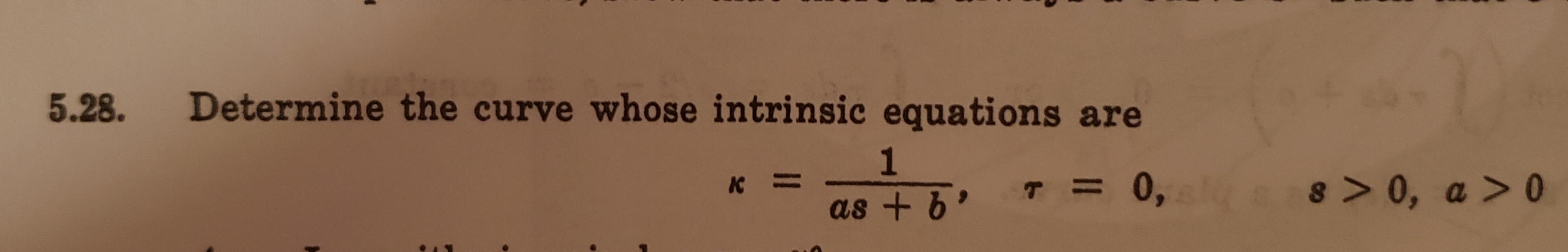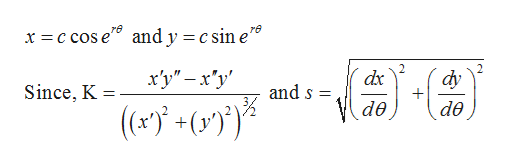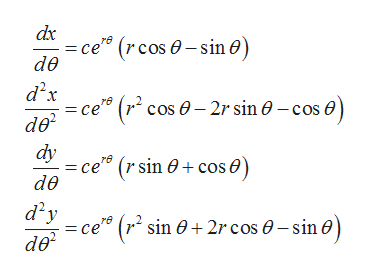# Determine the curve whose intrinsic equations are5.28.1a8 > 0, a >00,K11as +

Question
60 viewshelp_outlineImage TranscriptioncloseDetermine the curve whose intrinsic equations are 5.28. 1 a8 > 0, a >0 0, K 11 as + fullscreen
check_circle

Step 1

Given intrinsic equations are,

Step 2

Let the values of x and y are,help_outlineImage Transcriptionclosex -c cos e and y = c sin e" dy х'у" — х'у' dx and s = Since, K de de fullscreen
Step 3

Hence, the derivatives of x and y with ...help_outlineImage Transcriptionclosedx =ce (rcos0-sin 0) de d2x - ce (r cos 0-2r sin 0 - cos de2 dy = ce" de rв (r sin + cose) d2y ce (r sin 0+ 2rcos -sin de fullscreen

### Want to see the full answer?

See Solution

#### Want to see this answer and more?

Solutions are written by subject experts who are available 24/7. Questions are typically answered within 1 hour.*

See Solution
*Response times may vary by subject and question.
Tagged in

### Math